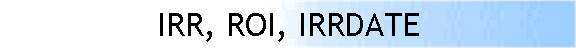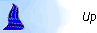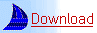In Excel the IRR (also known as ROI) can be calculated for a number of cash flows. For this calculation the assumption is that the cash flows happen only once per period. This is not always the case. Often enough there are multiple cash flows per period, and this requires that the user consolidates the data for each period before calculating the IRR. This can be a very tedious and time consuming activity that is prone to human error. We wrote a function (IRRDATE) that calculates the IRR only after it consolidates the cash flow data.DB Tools version 2.64 size 596 KB

This initial version only calculates by month, but future versions can also accommodate different periodic intervals.
In this function the first argument is the specified range. It must contain dates in the left column and values in the right column. The second argument allows you to enter the desired accuracy, the third allows you to specify how many digits will be displayed. Only the first argument is necessary for the function to calculate.  You  can see an example of what this function does (i.e. calculates) in the image below. The color coded arrows represent how the cash flows are consolidated by month.* Note: In the example the "months" are grouped around the last day of the month, i.e. the total March cash flow is determined using all cash flows from March 16 until April 15. In the example this provides the result that the April 2 cash flow really belongs to March.  You can specify a different cut-off (i.e. between March 1-31), with an optional argument that was added starting DB tools version 2.63.  If you need different intervals (i.e. quarterly, biannually, yearly), please drop us a line. We are interested in your ideas